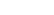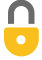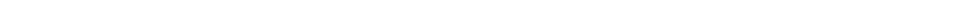Problem 27
Q

# What mass of steam at $100 ^\circ \textrm{C}$ must be added to 1.00 kg of ice at $0 ^\circ \textrm{C}$ to yield liquid water at $30 ^\circ \textrm{C}$?A
$0.18 \textrm{ kg}$In order to watch this solution you need to have a subscription.VIDEO TRANSCRIPT

This is Giancoli Answers with Mr. Dychko. The energy lost by the steam as it changes its phase into liquid and then also changes its temperature down to 30 degrees Celsius has to equal the heat gained by the ice as it changes its phase into liquid from solid and then increases its temperature to 30 degrees starting from 0. So, we'll solve this for mass of the steam required by taking this term to the right hand side. And... No, never mind. We're gonna be factoring out the ms, that's what's happening here. We factor out the ms and it becomes Lv plus specific heat of water times the initial temperature of the steam minus the final temperature. And then we're dividing both sides by that. And then we're isolating ms that way. So, mass of the steam is the mass of the ice which we can factor out from both of these two terms, times the latent heat of fusion because we're changing phase between solid and liquid here, so, that means we have latent heat of fusion here. Plus the specific heat of water because, you know, the ice now turns into water and then that water temperature has to increase up to 30 degrees starting from its initial temperature of 0. And then we're dividing that by the latent heat of vaporization because this is a phase change between gas and liquid for the steam. And then plus it's, you know, now water and so it's gonna have some heat lost. So, it's its initial temperature as 100 degrees Celsius minus its final temperature of 30. And then plugging numbers into all these things. Here's the latent heat of fusion for water 3.33 times 10 to the 5 joules per kilogram. And then latent heat of vaporization, 22.6 times 10 to the 5 joules per kilogram. It's 1 kilogram of ice that we start with. And I think I've explained all the other numbers there. And we end up with 0.18 kilograms of steam is needed to make all this happen.